# polynomial algorithm

## Finding k-edge-outerplanar graph embeddings ★★

Author(s): Bentz

Conjecture   It has been shown that a-outerplanar embedding for whichis minimal can be found in polynomial time. Does a similar result hold for-edge-outerplanar graphs?

Keywords: planar graph; polynomial algorithm

## Approximation ratio for k-outerplanar graphs ★★

Author(s): Bentz

Conjecture   Is the approximation ratio for the Maximum Edge Disjoint Paths (MaxEDP) or the Maximum Integer Multiflow problem (MaxIMF) bounded by a constant in-outerplanar graphs or tree-width graphs?

## Approximation Ratio for Maximum Edge Disjoint Paths problem ★★

Author(s): Bentz

Conjecture   Can the approximation ratiobe improved for the Maximum Edge Disjoint Paths problem (MaxEDP) in planar graphs or can an inapproximability result stronger than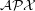-hardness?

## P vs. NP ★★★★

Author(s): Cook; Levin

Problem   Is P = NP?

## Subset-sums equality (pigeonhole version) ★★★

Author(s):

Problem   Letbe natural numbers with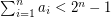. It follows from the pigeon-hole principle that there exist distinct subsetswith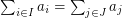. Is it possible to find such a pair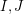in polynomial time?

Keywords: polynomial algorithm; search problem

## The stubborn list partition problem ★★

Author(s): Cameron; Eschen; Hoang; Sritharan

Problem   Does there exist a polynomial time algorithm which takes as input a graphand for every vertex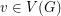a subsetof, and decides if there exists a partition ofinto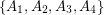so that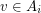only if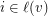and so that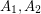are independent,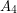is a clique, and there are no edges between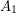and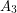?

Keywords: list partition; polynomial algorithm